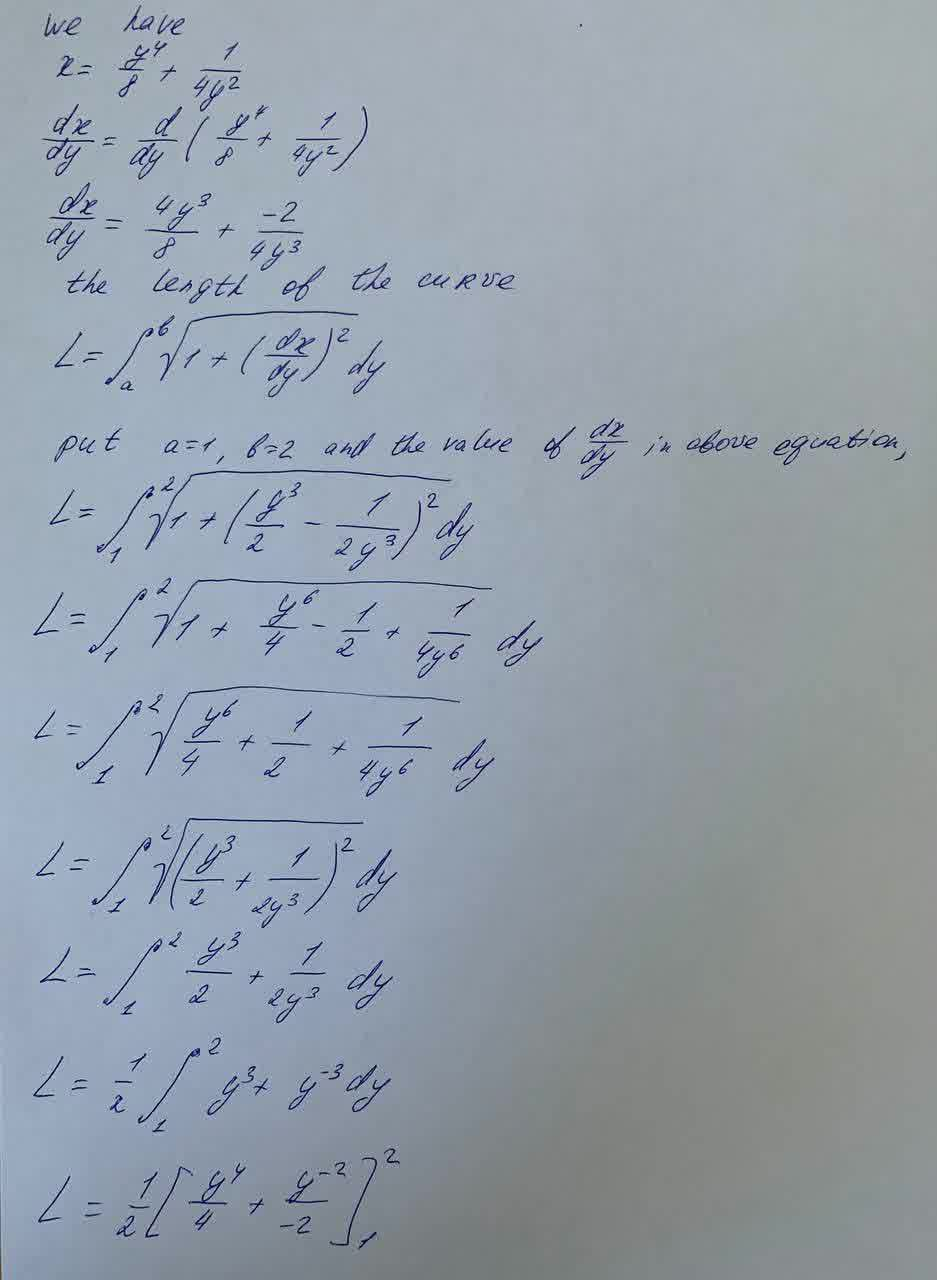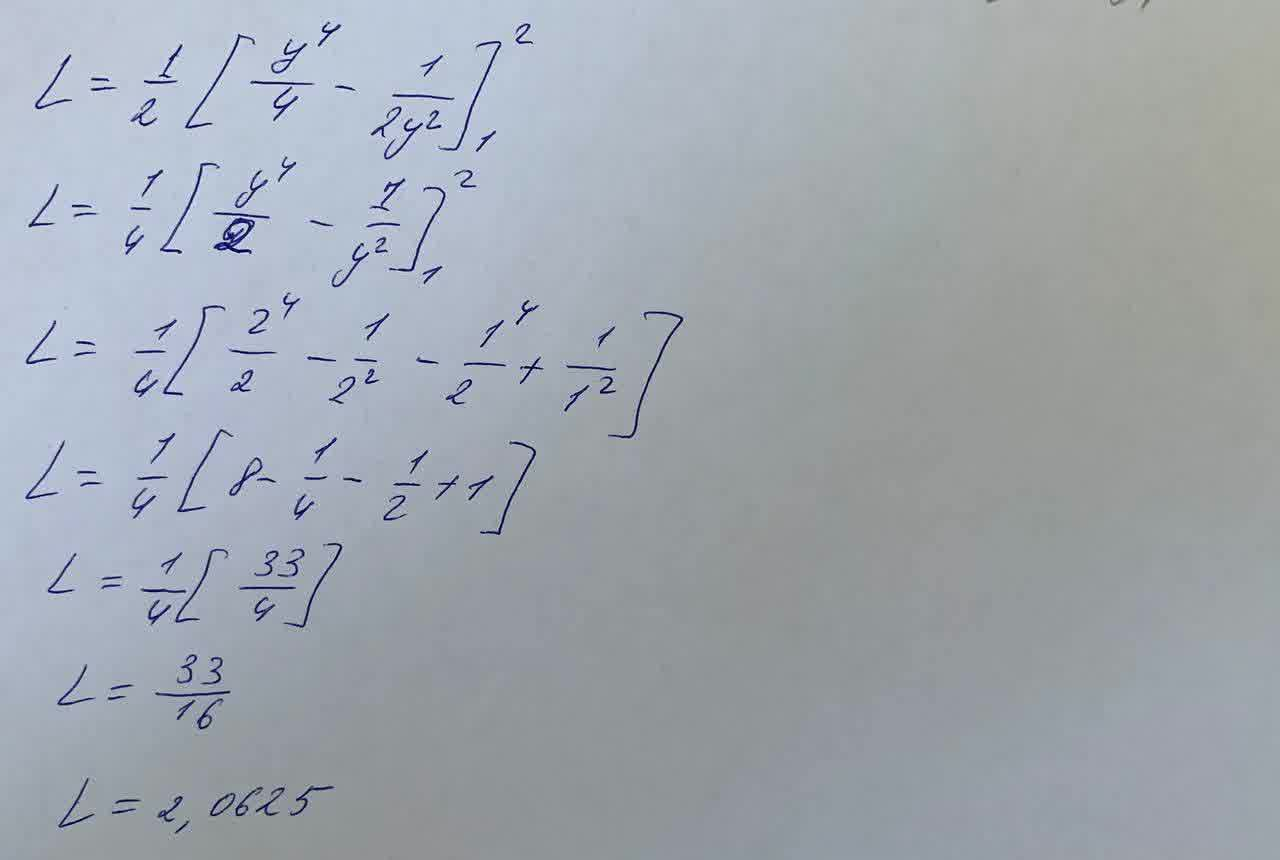Find the exact length of the curve. x = \frac{y^4}{8} + \frac{1}{4y^2} , 1 \leq y \leq 2Suman Cole 2021-06-03 Answered
Find the exact length of the curve. $$x = \frac{y^4}{8} + \frac{1}{4y^2} , 1 \leq y \leq 2$$

• Questions are typically answered in as fast as 30 minutes

Solve your problem for the price of one coffee

• Math expert for every subject
• Pay only if we can solve itArnold Odonnell